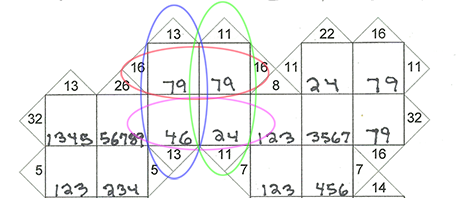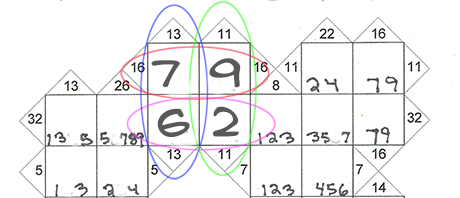Blog  |   Puzzles  |   Books  |   AboutI got a letter a few days ago, asking for help with a particularly intractable Kakuro puzzle: 8×8, Volume 14, Book 99, Puzzle #8. Since my Kakuro puzzles are arranged by difficulty, this is a particularly hard one, since it is in Book 99. Here you can see where the solver got stuck:I was able to make a little progress using an advanced technique I call “Implicit pairs”. Take a look at the 4 circled cells near the top of the puzzle.The vertical ovals show two containers, each containing a pair of cells, with the clues 13 and 11. The top horizontal oval is also a 2-cell container, with the clue 16. The bottom horizontal oval is around a pair of cells which are part of a much longer container.

Because we know the 4 cells sum to 13+11, we can work out the sum for the bottom two cells. It must be 13+11-16, or 8. So we can treat the bottom two cells as an “implicit pair” which sums to 8. Since it sums to 8, we can see that we can’t use the 4s, because 8 = 4+4, and this would cause a duplicate number. So we can eliminate the 4s, and this enables us to solve the four cells.Are you stuck on a Krazydad puzzle? Send me a snapshot and I’ll try to help!

Happy puzzling!